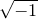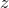# 4.5 Working with complex numbers

In all of the examples given thus far, algebraic expressions have only been allowed to return real numbers: Pyxplot has not been handling any complex numbers. Since there are many circumstances in which the data being analysed may be known for certain to be real, complex arithmetic is disabled in Pyxplot by default. Expressions such as sqrt(-1) will return either an error or NaN. The most obvious example of this is the built-in variable i, which is set to equal sqrt(-1):

pyxplot> print i
nan

Complex arithmetic may be enabled by typing

set numeric complex


and then disabled again by typing

set numeric real


Once complex arithmetic has been enabled, many of Pyxplot’s built-in mathematical functions accept complex input arguments, including the logarithm function, all of the trigonometric functions, and the exponential function. A complete list of functions which accept complex inputs can be found in Appendix 12.

Complex number literals can be entered into algebraic expressions in either of the following two forms:

print (2 + 3*i       )
print (2 + 3*sqrt(-1))


The former version depends upon the pre-defined system variable i being defined to equal. The user could cause this to stop working, of course, by re-defining this variable to have a different value. However, in this case the variable i could straightforwardly be returned to its default value by typing:

i=sqrt(-1)


The user can, of course, define any other variable to equal, thus allowing him to use any other letter, e.g. j, to represent the imaginary component of a number.

Several built-in functions are provided for manipulating complex numbers. The Re(z) and Im(z) functions return respectively the real and imaginary parts of a complex number. The arg(z) function returns the complex argument of. And the abs(z) function returns the modulus of. The conjugate(z) command returns the complex conjugate of. The following lines of code demonstrate the use of these functions:

pyxplot> set numeric complex
pyxplot> x=0.5
pyxplot> print Re(exp(i*x))
0.87758256
pyxplot> print cos(x)        # This equals the above
0.87758256
pyxplot> print arg(exp(i*x)) # This equals x
0.5